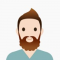## Maximum sum subsequence with at-least k distant elements in C++Published on 09-Sep-2020 13:30:59
In this tutorial, we will be discussing a program to find maximum sum subsequence with at-least k distant elements.For this we will be provided with an array containing integers and a value K. Our task is to find the subsequence having maximum sum such that all the elements are at ... Read More

## Maximum sum subarray such that start and end values are same in C++Published on 09-Sep-2020 13:29:00
In this tutorial, we will be discussing a program to find maximum sum subarray such that start and end values are same.For this we will be provided with an array containing integers. Our task is to find the subarray with the maximum sum such that the elements are both its ... Read More

## Maximum sum rectangle in a 2D matrix | DP-27 in C++Published on 09-Sep-2020 13:26:57
In this tutorial, we will be discussing a program to find maximum sum rectangle in a 2D matrix.For this we will be provided with a matrix. Our task is to find out the submatrix with the maximum sum of its elements.Example Live Demo#include<bits/stdc++.h> using namespace std; #define ROW 4 #define COL ... Read More

## Maximum sum possible for a sub-sequence such that no two elements appear at a distance < K in the array in C++Published on 09-Sep-2020 13:24:28
In this tutorial, we will be discussing a program to find maximum sum possible for a sub-sequence such that no two elements appear at a distance < K in the array.For this we will be provided with an array containing N intergers and a value K. Our task is to ... Read More

## Maximum sum path in a matrix from top to bottom in C++ ProgramPublished on 09-Sep-2020 13:22:05
In this tutorial, we will be discussing a program to find maximum sum path in a matrix from top to bottom.For this we will be provided with a matrix of N*N size. Our task is to find the maximum sum route from top row to bottom row while moving to ... Read More

## Maximum sum of smallest and second smallest in an array in C++Published on 09-Sep-2020 13:20:03
In this tutorial, we will be discussing a program to find maximum sum of smallest and second smallest in an array.For this we will be provided with an array containing integers. Our task is to find the maximum sum of smallest and second smallest elements in every possible iteration of ... Read More

## Maximum Sum of Products of Two Arrays in C++Published on 09-Sep-2020 13:18:27
In this tutorial, we will be discussing a program to find maximum Sum of Products of Two Arrays.For this we will be provided with two arrays of same size. Our task is to find the maximum sum by multiplying exactly one element from first element with one element from the ... Read More

## Maximum sum of pairwise product in an array with negative allowed in C++Published on 09-Sep-2020 13:16:30
In this tutorial, we will be discussing a program to find maximum sum of pairwise product in an array with negative allowed.For this we will be provided with an array containing integers. Our task is to find the maximum sum while performing pairwise multiplications.Example Live Demo#include <bits/stdc++.h> #define Mod 1000000007 using ... Read More

## Maximum sum of pairs with specific difference in C++Published on 09-Sep-2020 13:13:07
In this tutorial, we will be discussing a program to find maximum sum of pairs with specific difference.For this we will be provided with an array containing integers and a value K. Our task is to pair elements having difference less than K and finally find the maximum sum of ... Read More

## Maximum sum of nodes in Binary tree such that no two are adjacent in C++Published on 09-Sep-2020 13:10:07
In this tutorial, we will be discussing a program to find maximum sum of nodes in Binary tree such that no two are adjacent.For this we will be provided with a binary tree. Our task is to find the subset having maximum sum such that no two nodes in subset ... Read More